Question

# What would be the change in pressure in a sealed 10.0 L vessel due to the...

What would be the change in pressure in a sealed 10.0 L vessel due to the formation of N2 gas when the ammonium nitrite in 1.80 L of 1.00 M NH4NO2 decomposes at 25.0°C?

Here is the Solution: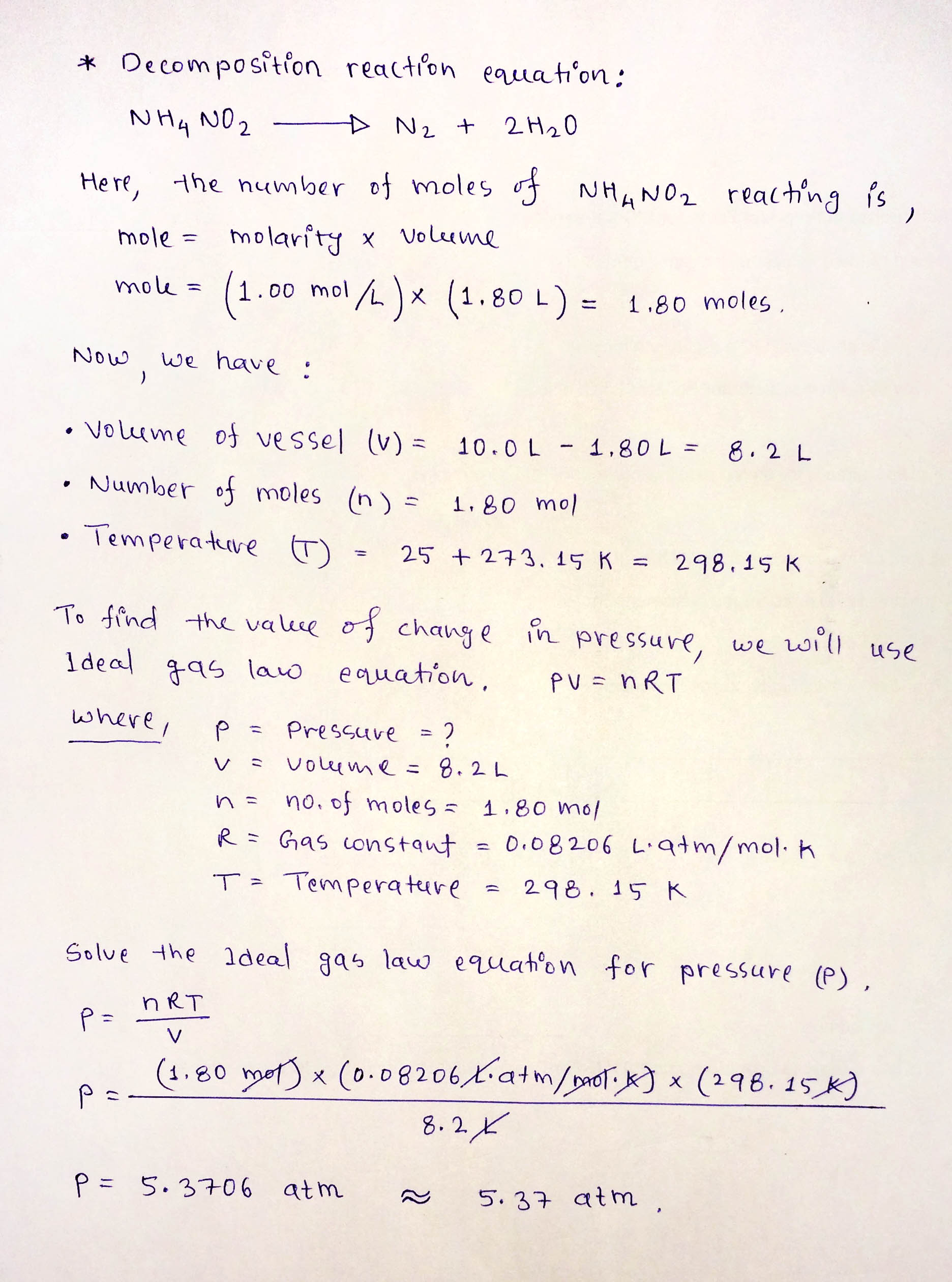Thanks! :-)

#### Earn Coins

Coins can be redeemed for fabulous gifts.

Similar Homework Help Questions
• ### What would be the change in pressure in a sealed 10.0 L vessel due to the...

What would be the change in pressure in a sealed 10.0 L vessel due to the formation of N2 gas when the ammonium nitrite in 2.40 L of 1.30 M NH4NO2 decomposes at 25.0°C?

• ### What would be the change in pressure in a sealed 10.0 L vessel due to the...What would be the change in pressure in a sealed 10.0 L vessel due to the formation of N2 gas when the ammonium nitrite in 2.00 L of 1.10 M NH4NO2 decomposes at 25.0°C? -5.3826 atm

• ### In some aquatic ecosystems, nitrate (NO3-) is converted to nitrite (NO2), which then decomposes to nitrogen...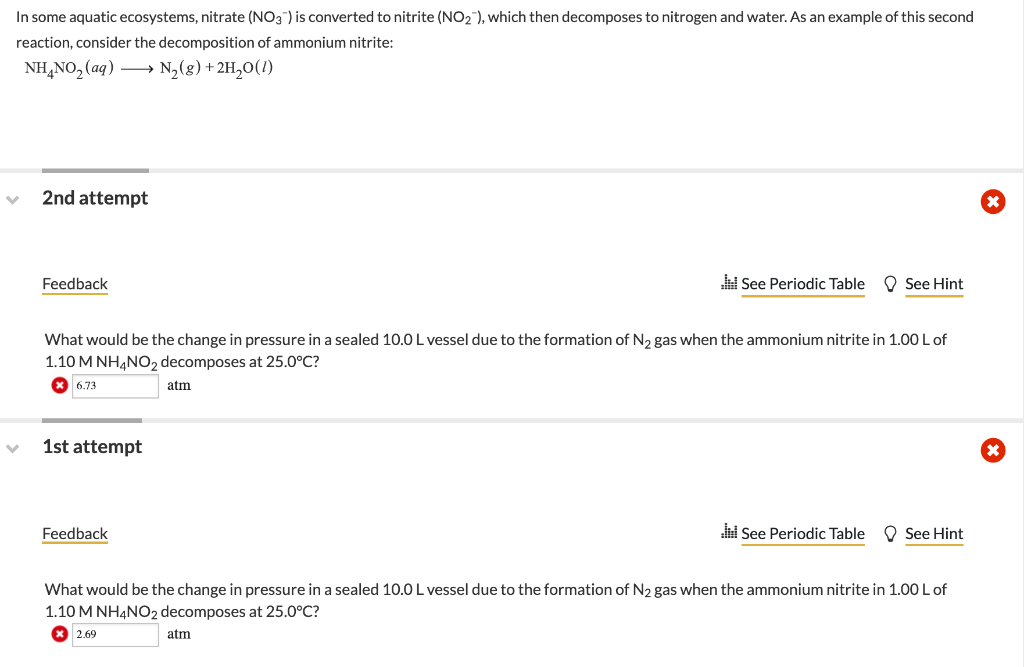In some aquatic ecosystems, nitrate (NO3-) is converted to nitrite (NO2), which then decomposes to nitrogen and water. As an example of this second reaction, consider the decomposition of ammonium nitrite: NH4NO2 (aq) →→N (8) +2H2O(1) 2nd attempt Feedback W See Periodic Table See Hint What would be the change in pressure in a sealed 10.0 L vessel due to the formation of N2 gas when the ammonium nitrite in 1.00 L of 1.10 M NH4NO2 decomposes at 25.0°C? ®...

• ### 19 Question (1 point) See page 293 In some aquatic ecosystems, nitrate (NO3) is converted to...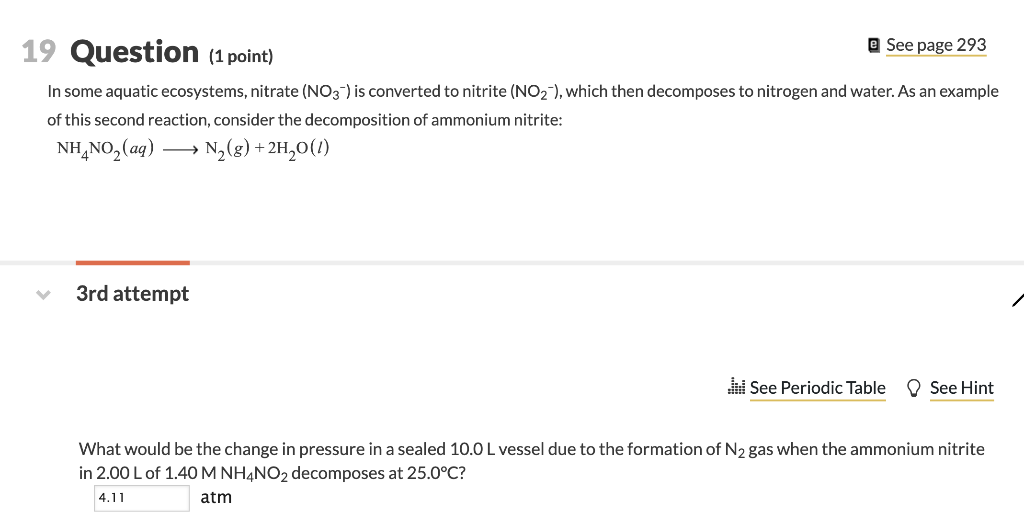19 Question (1 point) See page 293 In some aquatic ecosystems, nitrate (NO3) is converted to nitrite (NO2), which then decomposes to nitrogen and water. As an example of this second reaction, consider the decomposition of ammonium nitrite: NH NO2 (aq) N2(8)+2H20() 3rd attempt See Periodic Table See Hint What would be the change in pressure in a sealed 10.0 L vessel due to the formation of N2 gas when the ammonium nitrite in 2.00 L of 1.40M NH4NO2 decomposes...

• ### In some aquatic ecosystems, nitrate (NO3–) is converted to nitrite (NO2–), which then decomposes to nitrogen and water....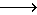In some aquatic ecosystems, nitrate (NO3–) is converted to nitrite (NO2–), which then decomposes to nitrogen and water. As an example of this second reaction, consider the decomposition of ammonium nitrite: \$\$NH4​NO2​(aq)N2​(g)+2H2​O(l) 3rd attempt See Hint See Periodic Table What would be the change in pressure in a sealed 10.0 L vessel due to the formation of N2gas when the ammonium nitrite in 2.60 L of 0.800 M NH4NO2 decomposes at 25.0°C?      atm   We were unable to transcribe this imageWe...

• ### 10 Question (1 point) See page 457 In some aquatic ecosystems, nitrate (NO3-) is converted to...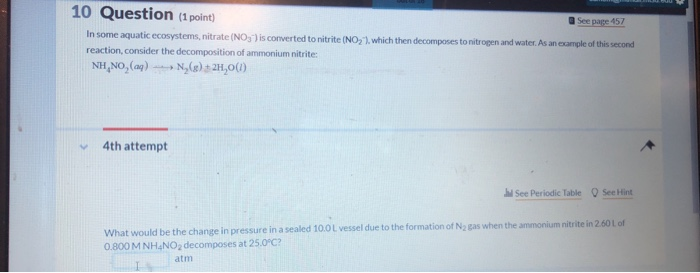10 Question (1 point) See page 457 In some aquatic ecosystems, nitrate (NO3-) is converted to nitrite (NO, which then decomposes to nitrogen and water. As an emple of this second reaction, consider the decomposition of ammonium nitrite: NH, NO, (a) N (8) + 2H,0(1) 4th attempt See Periodic Table See Hint What would be the change in pressure in a sealed 10.0L vessel due to the formation of N2 gas when the ammonium nitrite in 2.60L of 0.800 M...

• ### UL Jyll 2) When ammonium nitrite (NH4NO2) is heated, it decomposes to give nitro This property is used...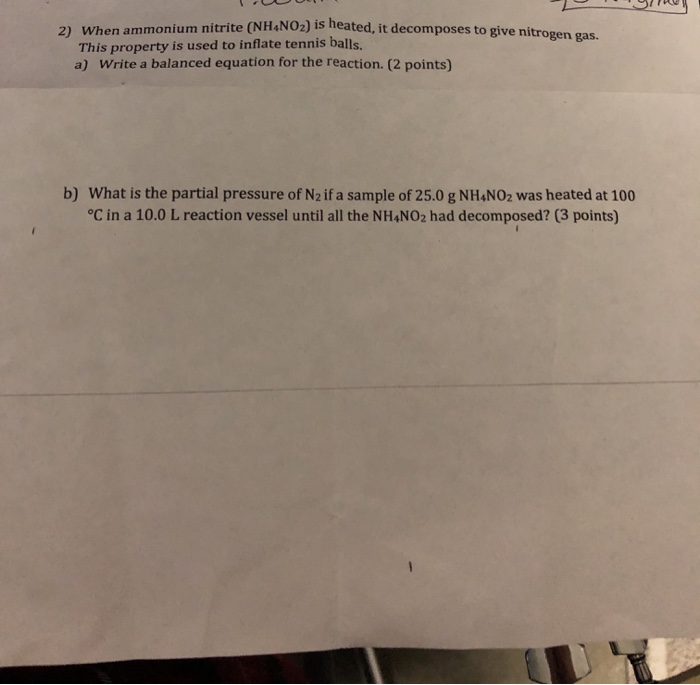UL Jyll 2) When ammonium nitrite (NH4NO2) is heated, it decomposes to give nitro This property is used to inflate tennis balls. a) Write a balanced equation for the reaction. (2 points) b) What is the partial pressure of N2 if a sample of 25.0 g NH4NO2 was heated at 100 °C in a 10.0 L reaction vessel until all the NH4NO2 had decomposed? (3 points) Calculate the quantity in grams y in grams of NH NO2 needed to inflate...

• ### Ammonium nitrite, NH4NO2, decomposes upon heating to form N2 gas according to the following balanced chemical...

Ammonium nitrite, NH4NO2, decomposes upon heating to form N2 gas according to the following balanced chemical equation. When a sample of NH4NO2 was decomposed in a test tube, 813.9 mL of N2 gas was collected over water at 47.64 °C and the total pressure was 765.9 torr. NH4NO2(s) → N2(g) + 2 H2O(l). How many grams of N2 were collected?

• ### CO2 (g) is placed in a sealed 5.0L vessel at 701 K and 10.0 atm. The...

CO2 (g) is placed in a sealed 5.0L vessel at 701 K and 10.0 atm. The vessel is heated to 1401K and the CO2 (g) partially decomposes to CO )g) and O2 (g). The final pressure in the vessel is 22.5 atm. a. What is the complete balanced equation for this reaction? b. How many moles of CO2 are present in the vessel initially (show work) c. How many moles are present in the flask at the end of the...

• ### Gas is contained in a 10.0 L vessel at a temperature of 40.0°C and a pressure...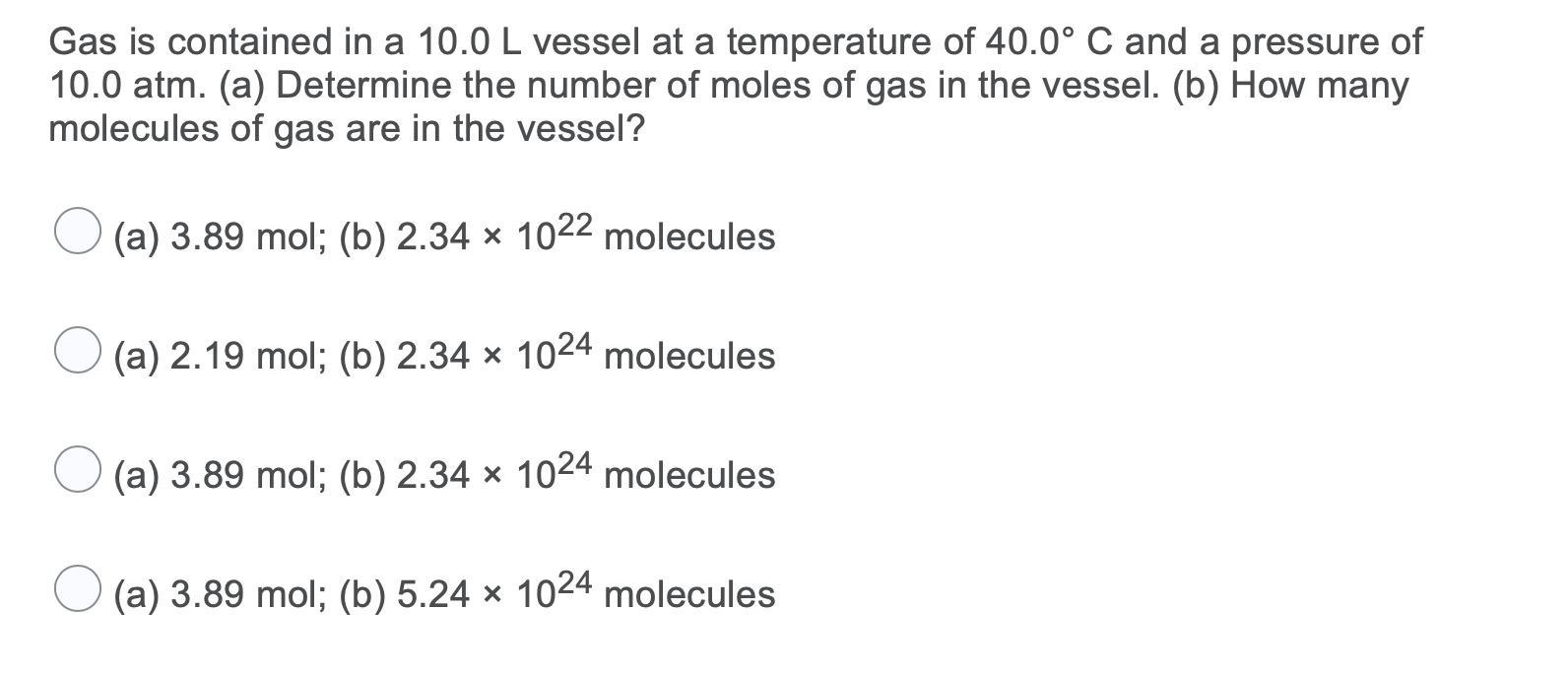Gas is contained in a 10.0 L vessel at a temperature of 40.0°C and a pressure of 10.0 atm. (a) Determine the number of moles of gas in the vessel. (b) How many molecules of gas are in the vessel? (a) 3.89 mol; (b) 2.34 * 1022 molecules (a) 2.19 mol; (b) 2.34 x 1024 molecules (a) 3.89 mol; (b) 2.34 x 1024 molecules (a) 3.89 mol; (b) 5.24 x 1024 molecules